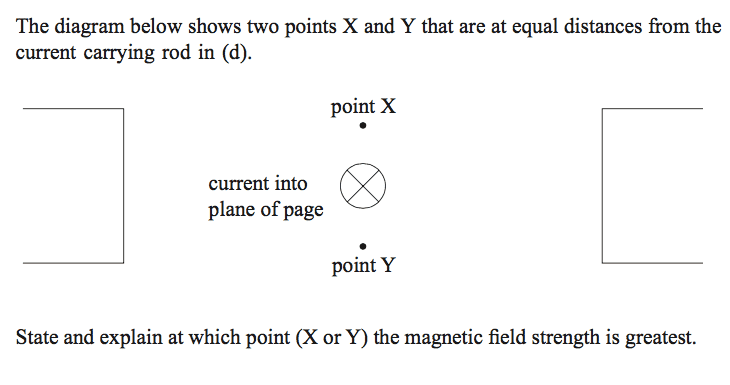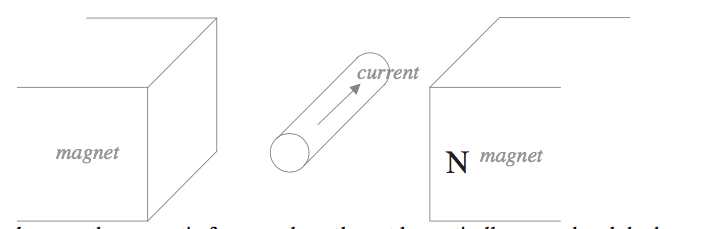# Magnetic Field Strength

## Homework Statement## The Attempt at a Solution

Shouldn't they be the same? Does one being above the current and the other being below make any difference? I know when there is a higher density of magnetic field lines, the magnetic field strength is greater, but in this case, isn't it the same?

## Answers and Replies

Mentor
I think you need to explain more about the scenario. What is the import of the (d) that is mentioned? What is the significance of the two truncated rectangles at wither side of the image? Is there a pre-existing magnetic field involved that you haven't mentioned? Please state the original question in its entirety.

I think you need to explain more about the scenario. What is the import of the (d) that is mentioned? What is the significance of the two truncated rectangles at wither side of the image? Is there a pre-existing magnetic field involved that you haven't mentioned? Please state the original question in its entirety.
Oh Yes, Sorry~Mentor
Okay, so there is an existing field due to the magnets represented by the rectangles. Sketch in the pre-existing field and then the field due to the current. Where do they reinforce and where do they oppose? You'll probably want to use the right and rule for the magnetic field due to a currentOkay, so there is an existing field due to the magnets represented by the rectangles. Sketch in the pre-existing field and then the field due to the current. Where do they reinforce and where do they oppose? You'll probably want to use the right and rule for the magnetic field due to a currentSo the pre-existing magnetic field is from right to left (N to S) , and then the field due to the current is clockwise...how do I determine where they reinforce?

Mentor
So the pre-existing magnetic field is from right to left (N to S) , and then the field due to the current is clockwise...how do I determine where they reinforce?
Sketch the vectors!